•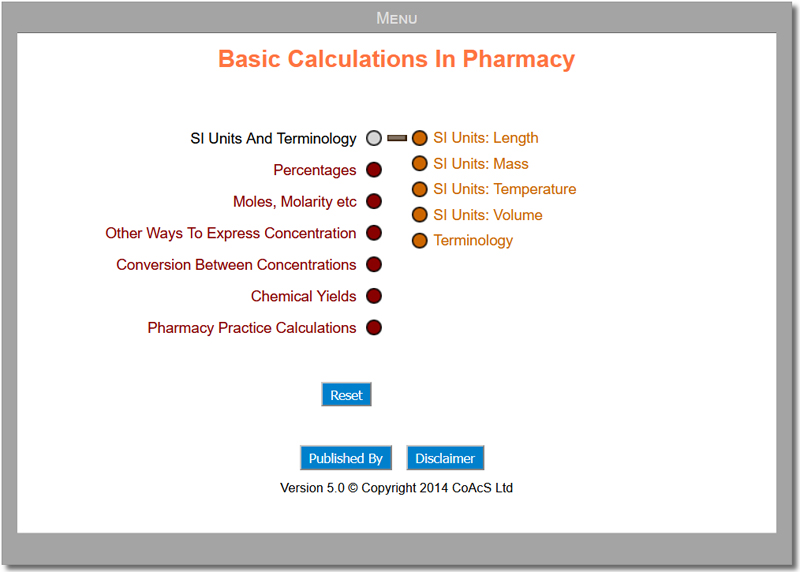•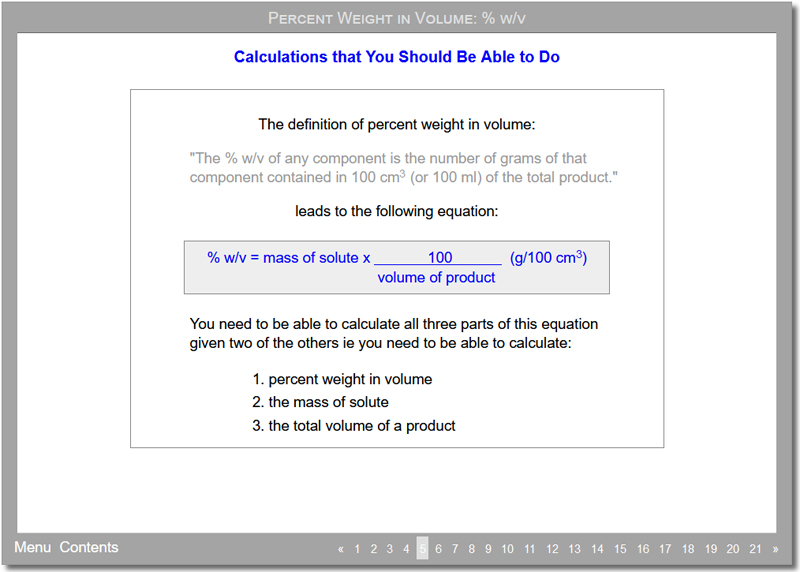•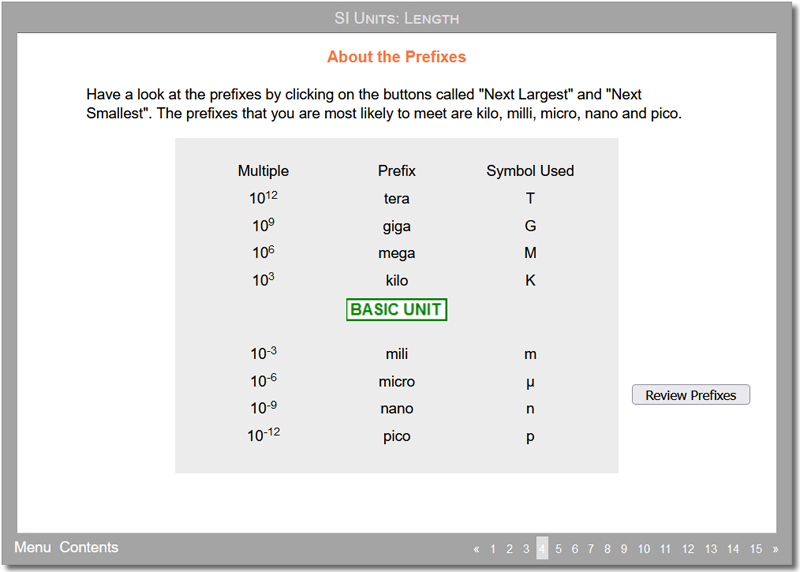•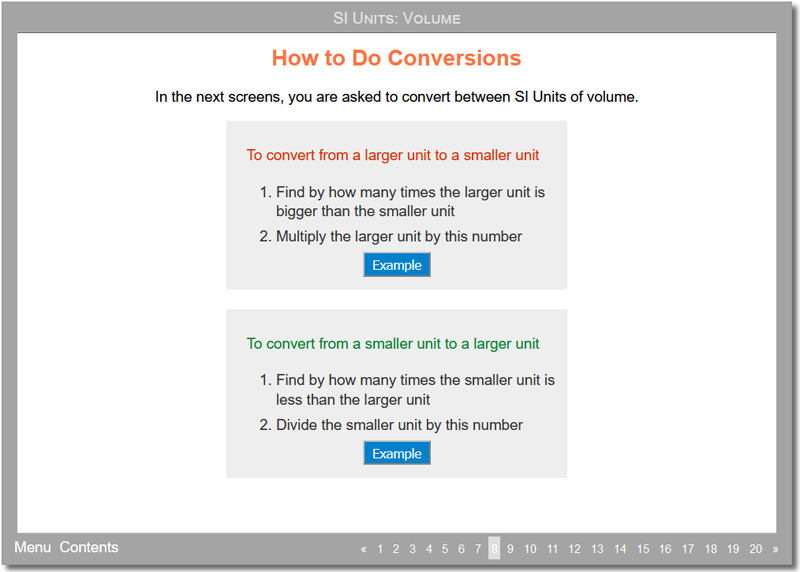•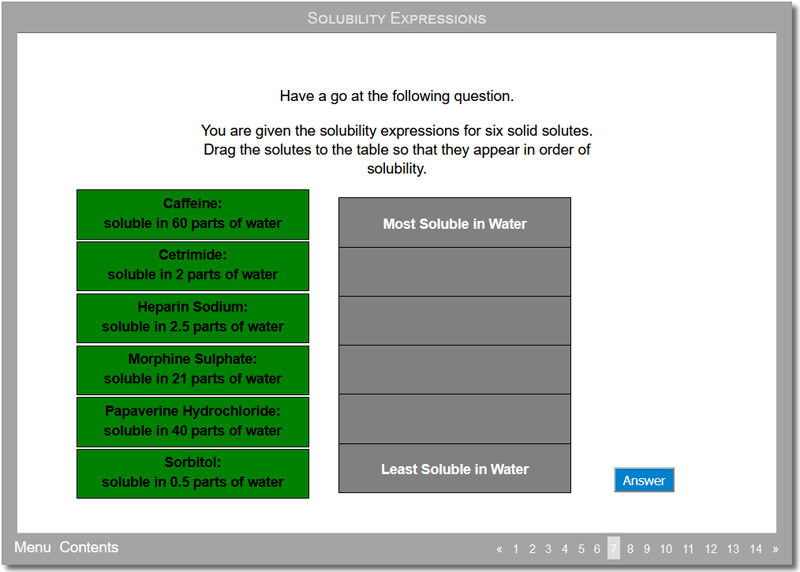•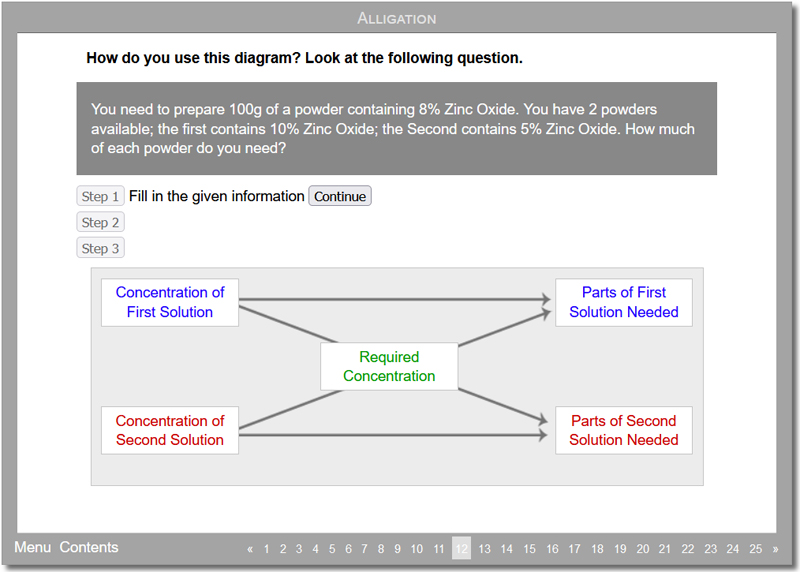•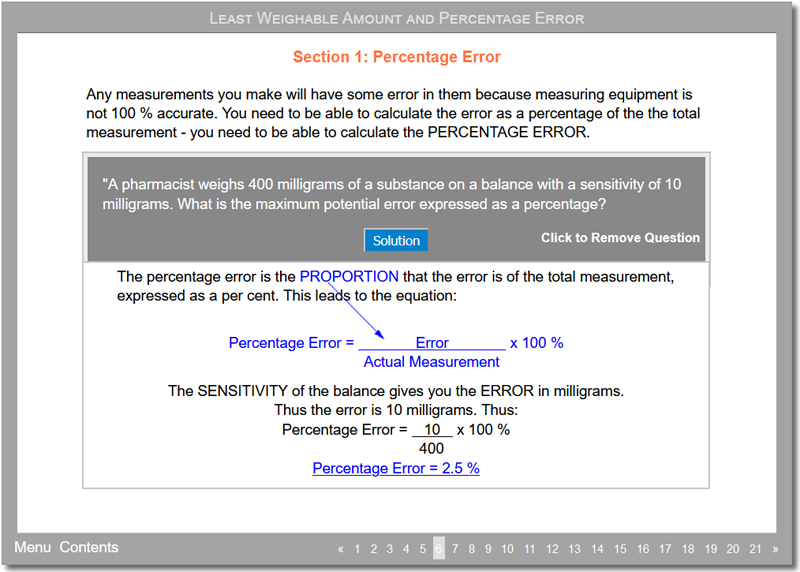•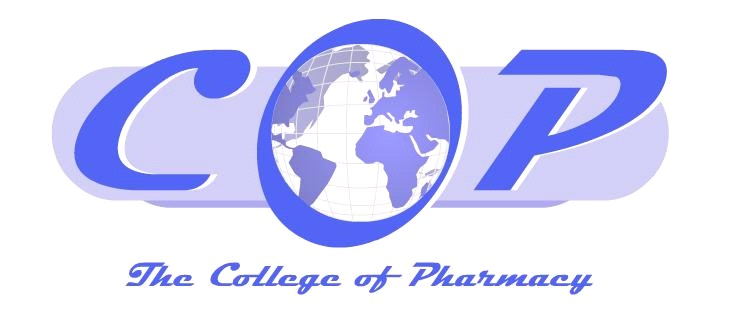(opens new window)

## Basic Calculations In Pharmacy

The package covers basic calculations and is intended to act as a bridge between school level and first year pharmacy degree level calculations. Those types of calculation covered in the package should already be known by the students; however experience has shown that this is not always the case.
The aims of the package are:

• To show the students the range of calculations that they are expected to know
• To provide help in those areas that students have commonly found problematical

Many of the activities within the package follow a similar structure:

• First, the student is given a brief introduction to the topic
• The student is then shown an equation that can be used for calculations
• For three-part equations the student then looks at how to calculate each part of the equation
• Finally, each activity finishes with a set of mixed questions

This package also contains an Assessment module with MCQs.

Product Code: COP001

### Price: £300.00 p.a. (per institution)

1: SI Units And Terminology

This is a basic subject introducing the student to the series of prefixes used for SI Units e.g. kilo-, deci-, nano- etc., the units which are used for measuring length, mass, temperature and volume and some of the terminology that the students might encounter. The subject consists of five activities:

• SI Units: Length
• SI Units: Mass
• SI Units: Temperature
• SI Units: Volume
• Terminology

2: Percentages

This subject introduces the student to ways of expressing concentration using percentages. Each activity shows the student a definition, uses of the concentration expression, how to prepare a solution of known concentration and an equation which can be used for calculations. The activity then gives example calculations and allows the student to practise questions. Two methods for solving the questions are shown: the first uses the equation itself, the second works from the definition and uses ratios more. The subject consists of four activities:

• Percentages
• Percent Weight in Volume
• Percent Weight in Weight
• Percent Volume in Volume

3: Moles, Molarity etc.

This subject introduces the student to ways to express concentration using moles. The activities – where appropriate – show the student how to prepare a solution of known concentration and an equation which can be used for calculations. The activities then show example calculations and allow the student to practise questions. The subject consists of four activities:

• Moles and RMM
• Molarity
• Mole Fraction
• Molality

4: Other Ways To Express Concentration

This subject looks at three other ways to express concentration other than those covered in the previous subjects. The subject consists of three activities:

• Parts Component and Ratios
• Solubility Expressions
• Normality and Equivalence

5: Conversions Between Concentrations

This subject contains a single activity called Test. The activity has no explanation and consists only of sets of questions testing whether the student can convert between the various ways to express concentration.

• Test

6: Chemical Yields

This subject has only one activity called Calculating Chemical Yields. In this activity, the student can find out how to calculate the theoretical yield of a reaction, the percentage yield of a reaction and the minimum amount of reactant needed to produce a specified amount of product.

• Calculating Chemical Yields

7: Pharmacy Practice Calculations

This subject covers some of the calculations that the student should be able to do if the student is to dispense chemicals correctly. There are six activities:

• Increasing/Decreasing Formulae
• Number of Doses
• Powder and Tablet Dilutions
• General Dilutions – Including Ointments (covering dilution of liquids and of solids)
• Using Stock Solutions (including Ethanol and Formaldehyde solutions)
• Alligation
• Least Weighable Amount / Percentage Error

Many of the activities within the package follow a similar structure:

First, the student is given a brief introduction to the topic:
For example in the topics on concentration, the student is given a definition of the concentration expression, told for what the concentration expression is used, how to prepare a solution etc. of a particular concentration and finally some of the problems that the student should be aware of when using that particular way of expressing concentration.

The student is then shown an equation that can be used for calculations:
Wherever possible, the student is shown how to derive the equation – often by using the definition given in the introduction.

For three-part equations the student then looks at how to calculate each part of the equation:
For each part of the equation the student follows an example calculation and can then have a go at some practice questions.

Finally, each activity finishes with a set of mixed questions:
These questions cover all the types of calculation covered in the activity and the questions are often harder and require the student to approach the problem in a slightly different way.

The package covers basic calculations and is intended to act as a bridge between school level and first year pharmacy degree level calculations. The types of calculation covered in the package should already be known by the students; however experience has shown that this is not always the case.

Pre-requisites: ‘A’ Level chemistry

Audience: Undergraduate pharmacy students and lecturers

Time to complete: 5 hours

“The calculations package offers a different, interactive and fun way to learn calculations related to pharmacy. The step-wise approach presented, facilitates the learning experience for students. A good number of calculations with different possible combinations are presented in each activity. The activities consist of an introduction, followed by some basic calculations related to the topic being covered, to ensure that the student has a good understanding of what is required. At the end of the activity, students are presented with a more challenging exercise to apply the knowledge learned.

Dr Nicolette Sammut Bartolo
B.Pharm.(Hons)(Melit.), M.Sc.(Melit.), Ph.D.(Melit.)
Lecturer
Department of Pharmacy
University of Malta

### Related resources

#### Main CoAcS websiteThe main CoAcS website explains more about the areas in which we operate including:

• pharmacy education
• healthcare applications to hospitals and pharma
• bespoke software
• pharmaceutical plant design and consultancy

#### College of Pharmacy (CoP)The College of Pharmacy (CoP) website is the gateway to all of our CAL packages. Besides the subscribed pharmacy learning materials, you will also have free access to the:

• museum – containing images of historical documents and pharmacy artifacts
• library – with listings of the latest pharmacy journals and other publications